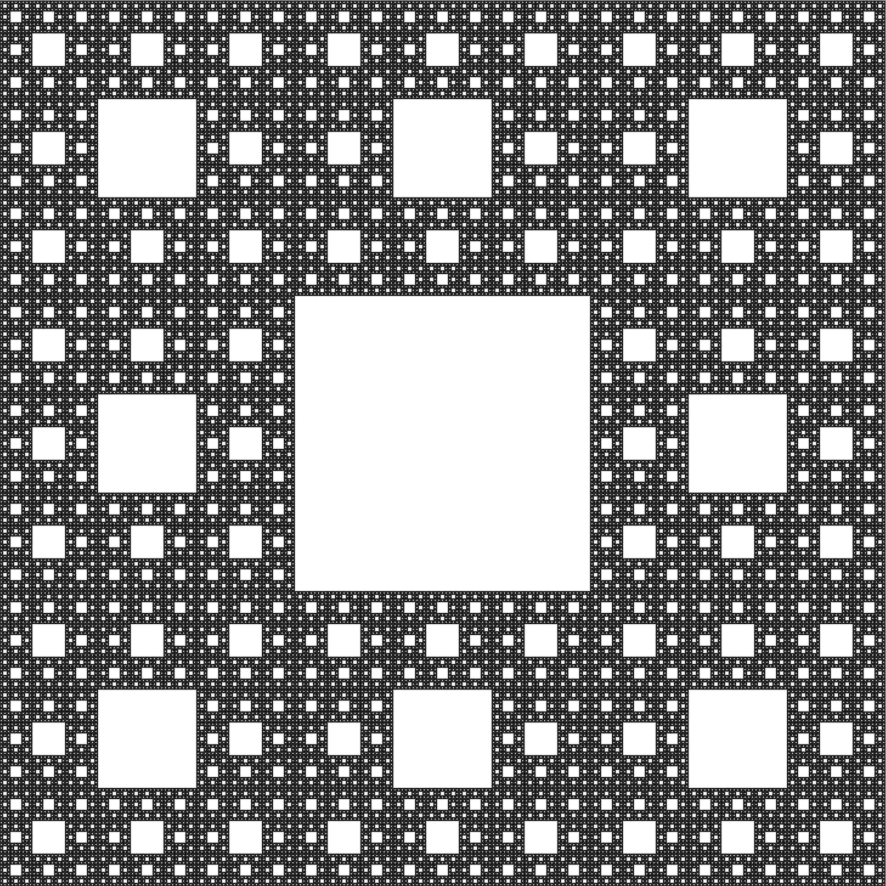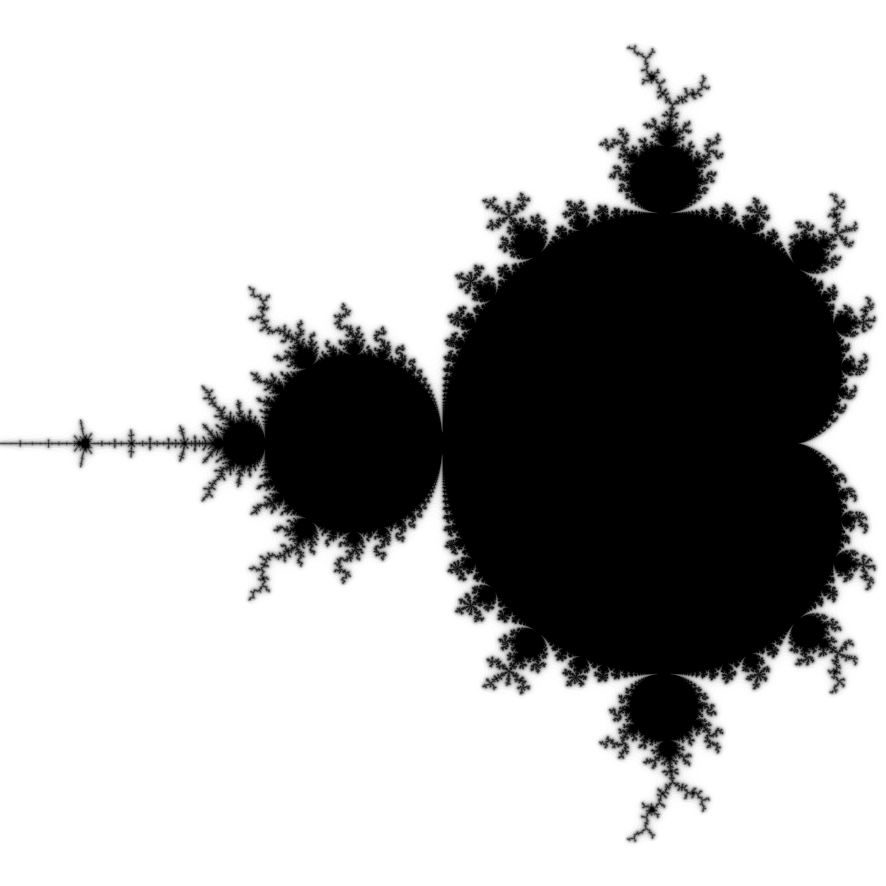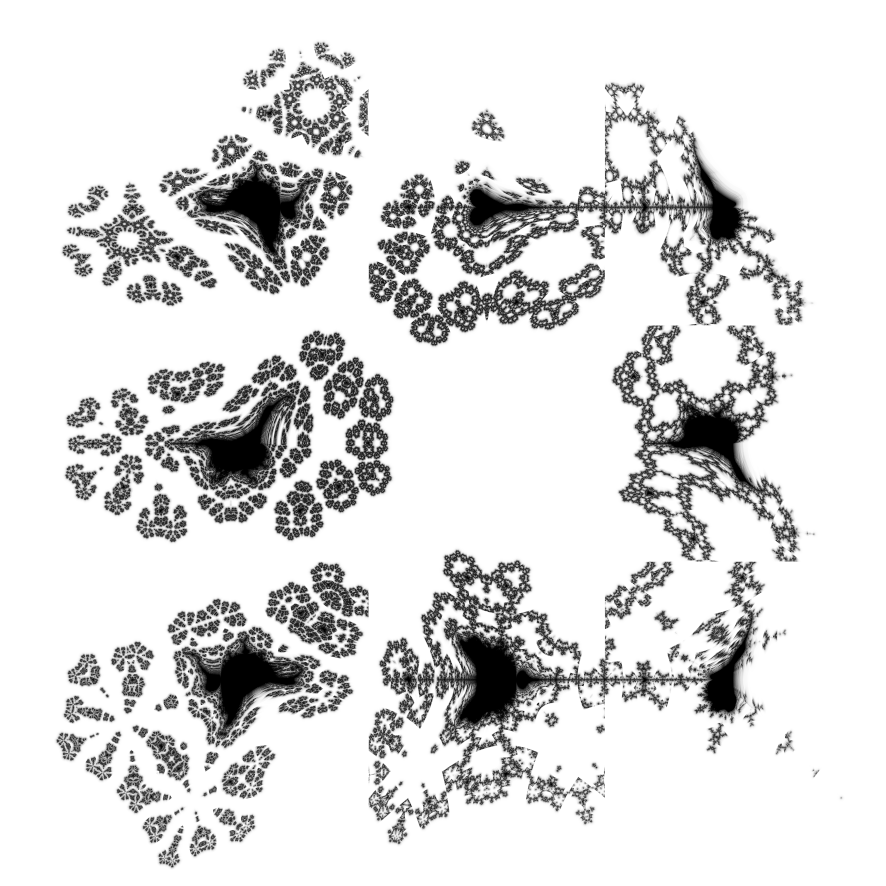# Distance Estimation for Hybrid Escape Time Fractals

2020-04-23

The well-known distance estimates for escape time fractals whose iteration behaviour near $$\infty$$ are like $$z \to a z$$ and like $$z \to z^p$$ are derived via the spatial derivative of a smooth iteration count. A distance estimate is derived for hybrid fractals with iteration behaviour like $$z \to a z^p$$.

# 1 The Sierpiński Carpet: $$z \to a z$$The Sierpiński carpet. Center $$0$$, zoom $$1$$.

The Sierpiński carpet is a figure consisting of $$8$$ copies of itself, each shrunk by a factor of $$\frac{1}{3}$$ and arranged around the border of a square (the central cell of the $$3 \times 3$$ grid is empty). The iterated function system for constructing the carpet can be turned into an escape time process (see knighty’s Kaleidoscopic Escape Time IFS1), whereby an input point $$z$$ is repeatedly transformed (including scaling by $$3$$ at each step) until $$z \to \infty$$.

The transformations have finite translations, so the behaviour near $$\infty$$ is like $$z \to 3 z$$ (the translations are insignificant). Fix a large $$R$$, and count the iterations $$n$$ where $$z_n$$ is the first iterate such $$R \le |z_n|$$. Start iterating from $$z_0$$ at the pixel coordinates.

Now $$R \le |z_n| < 3 R$$, because if $$3 R \le |z_n|$$ then $$R \le |z_{n-1}|$$ by the behaviour near $$\infty$$, which contradicts the assumption that $$z_n$$ is the first iterate to exceed $$R$$. The iteration count $$n$$ tells how quickly $$|z|$$ has exceeded $$R$$, and the size of $$|z|$$ at this iteration can be used to give a fractional iteration count: define $$f$$ by $$R \le |z_n| = 3^f R \le 3 R$$, then $$f = (\log|z_n| - \log R) / \log 3$$ and the smooth iteration count is $$m = n + 1 - f$$.

Approaching the Sierpiński carpet fractal boundary, the smooth iteration count increases without limit. The rate of increase is linked to the closeness to the boundary: an estimate of the distance is inversely proportional to the spatial derivative of the smooth iteration count. The spatial derivative of $$m = n + 1 - f$$ is equal to the spatial derivative of $$-f$$ almost everywhere (the steps between dwell bands are a set of measure zero), so $d = \frac{|z_n| \log 3}{\frac{\partial z_n}{\partial c}}.$(1.1)

For colouring images use $$d / \epsilon$$ where $$\epsilon$$ is the distance between neighbouring pixels: when this value is less than $$1$$ the pixel contains the fractal, when the value is greater than $$1$$ the pixel does not contain the fractal.

The same arguments apply in the general case when $$z \to a z$$ near $$\infty$$, with $$a > 1$$. The smooth iteration count is $m = n + 1 - \frac{\log|z_n| - \log R}{\log a},$(1.2) the renormalized smooth iteration count is derived by differentiating w.r.t. $$R$$, taking the limit as $$R \to \infty$$, then integrating again: $\mu = n - \frac{\log|z_n|}{\log a} + K$(1.3) where $$K$$ is an arbitrary constant of integration, and the distance estimate is $d = \frac{|z_n| \log a}{\frac{\partial z_n}{\partial c}}.$(1.4)

# 2 The Mandelbrot Set: $$z \to z^p$$The Mandelbrot set. Center $$-\frac{3}{4}$$, zoom $$\frac{4}{5}$$.

The Mandelbrot set $$M$$ is defined by iterations over the complex numbers $$\mathbb{C}$$ of $$z \to z^2 + c$$. It can be shown that if $$|z| > \max\left\{|c|,2\right\}$$ then $$z \to \infty$$, and a variant on the argument shows all $$|c| > 2$$ are outside $$M$$. Thus $$M$$ is finite.

The addition is small for $$c$$ near $$M$$, so the behaviour near $$\infty$$ is like $$z \to z^2$$ (the translation is insignificant). Fix a large $$R$$, and count the iterations $$n$$ where $$z_n$$ is the first iterate such that $$R \le |z_n|$$. Start the iterations from $$z_0 = 0$$.

Now $$R \le |z_n| < R^2$$, because if $$R^2 \le |z_n|$$ then $$R \le |z_{n-1}|$$ by the behaviour near $$\infty$$, which contradicts the assumption that $$z_n$$ is the first iterate to exceed $$R$$. The iteration count $$n$$ tells how quickly $$|z|$$ has exceeded $$R$$, and the size of $$|z|$$ at this iteration can be used to give a fractional iteration count: define $$f$$ by $$R \le |z_n| = R^{2^f} < R^2$$, then $$f = \log(\log|z_n| / \log R) / \log 2$$ and the smooth iteration count is $$m = n + 1 - f$$.

The renormalized smooth iteration count is derived by differentiating w.r.t. $$R$$, taking the limit as $$R \to \infty$$, then integrating again: $\mu = n - \frac{\log \log|z_n|}{\log 2} + K$(2.1) where $$K$$ is an arbitrary constant of integration.

Approaching the Mandelbrot set fractal boundary, the smooth iteration count increases without limit. The rate of increase is linked to the closeness to the boundary: an estimate of the distance is inversely proportional to the spatial derivative of the smooth iteration count. The spatial derivative of $$\mu = n - g + K$$ is equal to the spatial derivative of $$-g$$ almost everywhere (the steps between dwell bands are a set of measure zero), so the distance estimate is $d = \frac{|z_n| \log |z_n| \log 2}{\frac{\partial z_n}{\partial c}}.$(2.2)

For colouring images use $$d / \epsilon$$ where $$\epsilon$$ is the distance between neighbouring pixels: when this value is less than $$\frac{1}{2}$$ the pixel contains the fractal, when the value is greater than $$2$$ the pixel does not contain the fractal. The factor of $$4$$ between these two figures is due to the Koebe $$\frac{1}{4}$$ theorem, which bounds the distortion of conformal maps, detailed discussion is beyond the scope of this document. See Yumei Dang, Louis H. Kauffman and Dan Sandin, “Hypercomplex Iterations: Distance Estimation and Higher Dimensional Fractals”2.

The same arguments apply in the general case when $$z \to z^p$$ near $$\infty$$, with $$p > 1$$. The smooth iteration count is $m = n + 1 - \frac{\log\frac{\log|z_n|}{\log R}}{\log p},$(2.3) the renormalized smooth iteration count is $\mu = n - \frac{\log \log|z_n|}{\log p} + K,$(2.4) and the distance estimate is $d = \frac{|z_n| \log |z_n| \log p}{\frac{\partial z_n}{\partial c}}.$(2.5)

# 3 Hybrid Fractals: $$z \to a z^p$$Mandelbrot set / Sierpiński carpet hybrid. The Sierpiński carpet is implemented with $$|\cdot|$$ folding. Center $$0$$, zoom $$\frac{4}{5}$$.

One can make a hybrid of two fractals by interleaving their iteration steps. A hybrid of a fractal that escapes like $$z \to a z$$ with a fractal that escapes like $$z \to z^p$$ will escape like $$z \to a z^p$$.

Fix a large $$R$$, and count the iterations $$n$$ where $$z_n$$ is the first iterate such that $$R \le |z_n|$$. Start the iterations from $$z_0 = 0$$ and interleave the exponential iterations first with the multiplicative iterations second.

Now $$R \le |z_n| < aR^p$$, because if $$aR^p \le |z_n|$$ then $$R \le |z_{n-1}|$$ by the behaviour near $$\infty$$, which contradicts the assumption that $$z_n$$ is the first iterate to exceed $$R$$. The iteration count $$n$$ tells how quickly $$|z|$$ has exceeded $$R$$, and the size of $$|z|$$ at this iteration can be used to give a fractional iteration count.

Unfortunately defining $$f$$ by the simple $$R \le |z_n| = a^f R^{p^f} < aR^p$$ does not work as it isn’t smooth. One can see this by differentiating with respect to $$f$$: $\frac{\partial}{\partial f} a^f R^{p^f} = a^f R^{p^f} \left(\log a + p^f \log p \log R\right)$(3.1) Setting $$f = 1$$ now gives a different value to setting $$f = 0$$ after making the substitution $$R \to a R^p$$.

By Schröder 1870, a smooth interpolant is $R \le |z_n| = a^\frac{p^f-1}{p-1} R^{p^f} < a R^p$(3.2) Solving for $$f$$ gives $f = \log\left(\frac{\log a + (p - 1) \log |z_n|}{\log a + (p - 1) \log R}\right) / \log p$(3.3) and the smooth iteration count is $$m = n + 1 - f$$. The renormalized smooth iteration count is found by differentiating w.r.t. $$R$$, taking the limit as $$R \to \infty$$ before integrating again: $\mu = n - \frac{\log (\log a + (p - 1) \log |z_n|)}{\log p} + K$(3.4) where $$K$$ is an arbitrary constant of integration. As before, the distance estimate is inversely proportional to the spatial derivative: $d = \frac{|z_n| \log p \left(\log a + (p - 1) \log |z_n| \right)}{\frac{\partial z_n}{\partial c} (p-1)}$(3.5)

If it is known that an iterate escapes like $$z \to a z^p$$ but the values of the constants are not known, they can be estimated numerically by performing two more iterations after escape:

$p = \frac{\log |z_{n+2}| - \log |z_{n+1}|}{\log |z_{n+1}| - \log |z_n|}$(3.6) $\log a = \log |z_{n+1}| - p \log |z_n|$(3.7)

# 4 Generalizations

The maths carries through without any difficulty to higher-dimensional Menger sponge, Mandelbulb, etc. Note that non-(complex)-analytic functions need general Jacobian matrices of derivatives rather than a single (complex) derivative as used for the Mandelbrot set. A convenient implementation technique is using vectors of extended dual numbers (with $$2$$ or more orthogonal dual parts corresponding to the dimension of the space) for automatic differentiation.

The form $$z \to a z^p$$, together with the earlier special forms for $$p = 1$$ and $$a = 1$$, are general enough to handle all polynomial iterations, because near $$\infty$$ the highest power term dominates. Hybrids of multiple polynomial iterations are polynomial iterations themselves: for example if $$A : z \to z^p$$ and $$B : z \to a z$$ then $$B \circ A : z \to a z^p$$, $$A \circ B : z \to a^p z^p$$, $$B \circ A \circ A : z \to a z^{2p}$$, $$B \circ B \circ A : z \to a^2 z^p$$, and so on. Any eventually-repeating hybrid can be constructed, provided that the escape radius $$R$$ is large enough compared with the domain of evaluation (the region of $$c$$ values) such that the non-repeating part is over by the time the first $$z_n$$ escapes.

# 5 Questions

## 5.1 The $$\log a$$ Factor

The distance estimate derived here for $$z \to a z$$ differs from some of the distance estimates in the implementations3 by a factor of $$\log a$$.

## 5.2 The $$\log p$$ Factor

The distance estimate derived here for $$z \to z^p$$ differ from some of the distance estimates in the implementations and literature by a factor of $$\log p$$.

The distance estimate for the quadratic Mandelbrot set is apparently4 $d = \frac{1}{\frac{\partial \mu}{\partial c} \log 2}$(5.1) which works out to $d = \frac{|z_n| \log |z_n|}{\frac{\partial z_n}{\partial c}}$ with the $$\log 2$$ cancelled compared to Equation 2.2.

## 5.3 Upper and Lower Bounds

For a sphere marching ray tracer, one needs a lower bound on the distance. If the distance is ever overestimated, overstepping badness results. The distance estimates derived here are only approximate, not strict bounds.

The Hypercomplex book chapter 3.6 has a lower bound for the distance of a point $$z$$ to a quadratic Julia set $$K_c$$ of: $d(z, K_c) > \frac{\sinh{G(z)}}{2\exp{G(z)} |G'(z)|} \approx \frac{1}{2|z_n|^\frac{1}{2^n}} \frac{|z_n| \log |z_n|}{|z_n'|}$(5.2) whose extra factor of $$|z_n|^\frac{1}{2^n} \to 1+$$ as $$n \to \infty$$, for $$\mu > 7$$ the error from ignoring this term is less than 1%, but it may need to be taken into account further from the fractal.

## 6.1 Renormalizing the Mandelbrot Escape

The scale-dependence on the escape radius can be (partly) removed by differentiating the above formula with respect to the escape radius, taking the limit to infinity, and then integrating the first term of the resulting differential equation. The result is a renormalized, scale-independent, quasi-integral-valued iteration count. – Linas Vepstas (1997) “Renormalizing the Mandelbrot Escape”5

## 6.2 M-Set Derivatives

The “distance estimator” is the inverse infinitesimal flow of the iteration number. It gets this name because it provides a rough estimate of how far away an exterior point is from the boundary of the M-Set. The smooth (real-valued) iteration count is given by $$\mu = n+1-\log(\log(|z|))/\log 2$$, as demonstrated in the Escape Theory Room (which is, in turn, the logarithm of the Douady-Hubbard potential). Taking the derivative of $$\mu$$ w.r.t. $$c$$ we get $$d\mu/dc = d|z|/dc / (|z| \log |z| \log 2)$$. The “distance estimator” is one over this quantity. – Linas Vepstas (1997, 2000) “M-Set Derivatives”6

## 6.3 Ueber iterirte Functionen

Setzt man z.B.: $\phi(z) = a z^n,$(6.1) so findet man leicht direct: $\phi^r(z) = a^\frac{n^r-1}{n-1} z^{n^r}.$(6.2) – Ernst Schröder (1870). “Ueber iterirte Functionen”. Math. Ann. 3 (2): 296–322. doi:10.1007/BF01443992.7